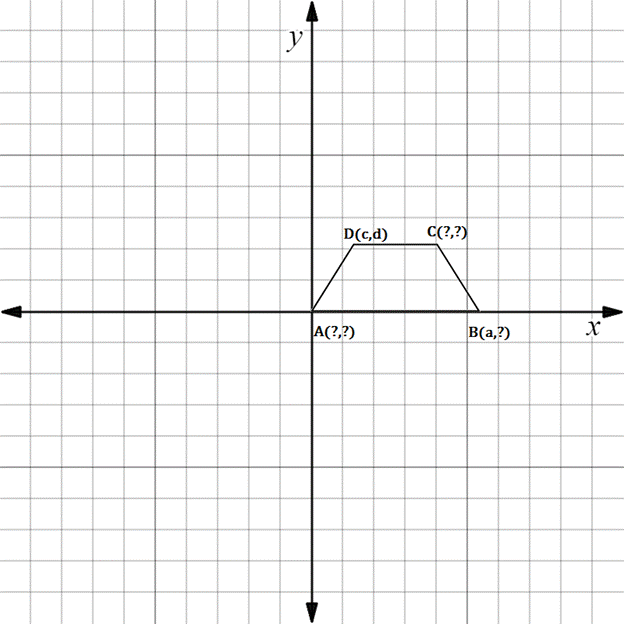Chapter 10.3, Problem 15EElementary Geometry For College St...

7th Edition
Alexander + 2 others
ISBN: 9781337614085

Solutions

Chapter
SectionElementary Geometry For College St...

7th Edition
Alexander + 2 others
ISBN: 9781337614085
Textbook Problem

In Exercises 11 to 16, supply the missing coordinates for the vertices, using as few variables as possible.A B C D is an isosceles trapezoid; A B - ║ D C - and A D - ≅ B C - .

To determine

To find:

The missing coordinates for the vertices.

Explanation

The given figure is shown below.

From the figure, the following observations are made.

Since, the vertex A is at the origin.

The coordinates of A0, 0.

Now, consider the vertex B.

Here, x-coordinate is given as a.

In the B vertex, observe where it lies on y-axis.

Since, it is at 0, the vertex B has the coordinates a, 0.

Consider the vertex C

Still sussing out bartleby?

Check out a sample textbook solution.

See a sample solution

The Solution to Your Study Problems

Bartleby provides explanations to thousands of textbook problems written by our experts, many with advanced degrees!

Get Started